##### TI-84 Plus CE Graphing Calculator For Dummies, 3rd EditionYour TI-84 Plus calculator has a CMPLX menu of functions designed to accomplish just about any task you need to when working with complex numbers.

The functions most often used with complex numbers are all located in one convenient location on your calculator. To access the CMPLX menu, press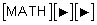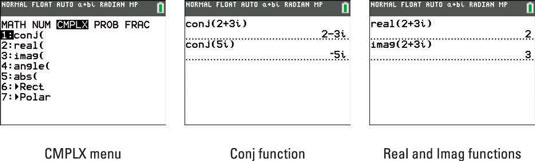## Finding the conjugate of a complex number

Finding the conjugate of a complex number is so easy that you probably don’t need a calculator for the task. In case you do, press  on the CMPLX menu to use the Conj function. Enter the expression you want to find the conjugate of and press [ENTER]. See the second screen.

## Finding the real and imaginary parts of a complex number

This is another function that seems to do the obvious, indicating they are mainly used in programming. In the CMPLX menu, press  to insert the Real function or press  to insert the Imag function. Enter a complex number in the argument and press [ENTER] to see your predictable results shown in the third screen. These tools simply identify the real or imaginary part of a complex number.

Before proceeding, press [MODE] and make sure your calculator is in RADIAN mode. Generally speaking, this is the recommended mode for all complex number calculations.

## Finding the polar angle of a complex number

The Angle function uses the formula tan1(b/a) to calculate the polar angle of a complex number (where a is the real part and b is the imaginary part). In the CMPLX menu, press  to insert the Angle function, type a complex number in the argument, and then press [ENTER]. See the first screen.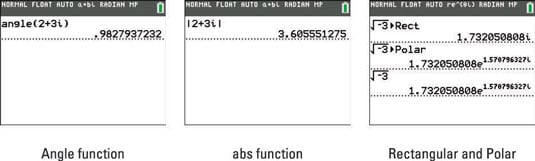## Finding the magnitude (modulus) of a complex number

Entering a complex number in the absolute value template finds the magnitude (modulus) of the complex number. Algebraically your calculator uses the formula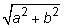for the calculation. In the CMPLX menu, press  to access the abs function, type a complex number, and then press [ENTER]. See the second screen.

Press [ALPHA][WINDOW] to access the abs function in the shortcut menu.

## Displaying a complex result in polar or rectangular form

The last two choices in the CMPLX menu work only when inserted after typing a complex number. Use Rect to display a complex number in rectangular form. To display a complex number in polar form, select Polar from the CMPLX menu. See the first two lines of the last screen.

Save time converting complex numbers to polar form by changing the mode of your calculator to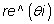As shown in the last line of the last screen, simply type a complex number and press [ENTER] to convert to polar form!